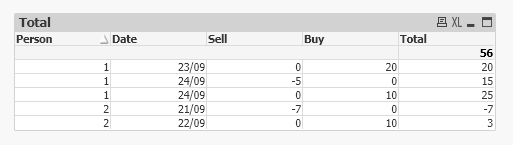# QlikView App Dev

Discussion Board for collaboration related to QlikView App Development.

Announcements
Live chat with experts, bring your API Integration questions. June 15th, 10 AM ET. REGISTER TODAY
cancel
Showing results for
Search instead for
Did you mean:Contributor

## Calculating rows on a table

Hi,

I'm trying to write a formula where I'm calculating the values on various rows depending on the person that is showing in the table and by the date - so for example:

Person  Buy            Sell  Date
1               10                         24/09
1                                   -5      24/09
1               20                         23/09
2               10                         22/09
2                                  -7       21/09

so then I would have a 5th column called Total and within each row there will be a breakdown of accumulation of the buy/sell for each person.

The table would look like below:
Person  Buy          Sell  Date             Total
1               10                           24/09            25
1                                   -5        24/09           15
1               20                           23/09           20
2               10                           22/09            3
2                                  -7         21/09           -7

I've tried a few things but it just seems to give me an overall value - does anyone know how I could achieve this?
Any help is much appreciated!

Labels (1)
• ### QlikView

1 Solution

Accepted SolutionsMVP

@mckay9999  try below. Assuming that your Date field is in proper Date format so that  order by statement can work as expected. If it is not in proper date format then try to convert it first.

``````Data:
LOAD Person,
Date,
if(len(trim(Buy))=0,0,Buy) as Buy,
if(len(trim(Sell))=0,0,Sell) as Sell
FROM Table:

Final:
LOAD *,
if(Person=Peek(Person), rangesum(peek(Buy_accum),Buy),Buy) as Buy_accum,
if(Person=Peek(Person),rangesum(peek(Sell_Accum),Sell),Sell) as Sell_Accum
Resident Data
order by Person,Date,Buy;

DROP Table Data;``````

You can then use below expression in chart

``=sum(Buy_accum)+sum(Sell_Accum)``3 RepliesMVP

@mckay9999  do you want to do it in script or frond end?Contributor
Author

Hi Kush,

Thanks for the reply - within the load script if that is possible please?

ThanksMVP

@mckay9999  try below. Assuming that your Date field is in proper Date format so that  order by statement can work as expected. If it is not in proper date format then try to convert it first.

``````Data:
LOAD Person,
Date,
if(len(trim(Buy))=0,0,Buy) as Buy,
if(len(trim(Sell))=0,0,Sell) as Sell
FROM Table:

Final:
LOAD *,
if(Person=Peek(Person), rangesum(peek(Buy_accum),Buy),Buy) as Buy_accum,
if(Person=Peek(Person),rangesum(peek(Sell_Accum),Sell),Sell) as Sell_Accum
Resident Data
order by Person,Date,Buy;

DROP Table Data;``````

You can then use below expression in chart

``=sum(Buy_accum)+sum(Sell_Accum)``